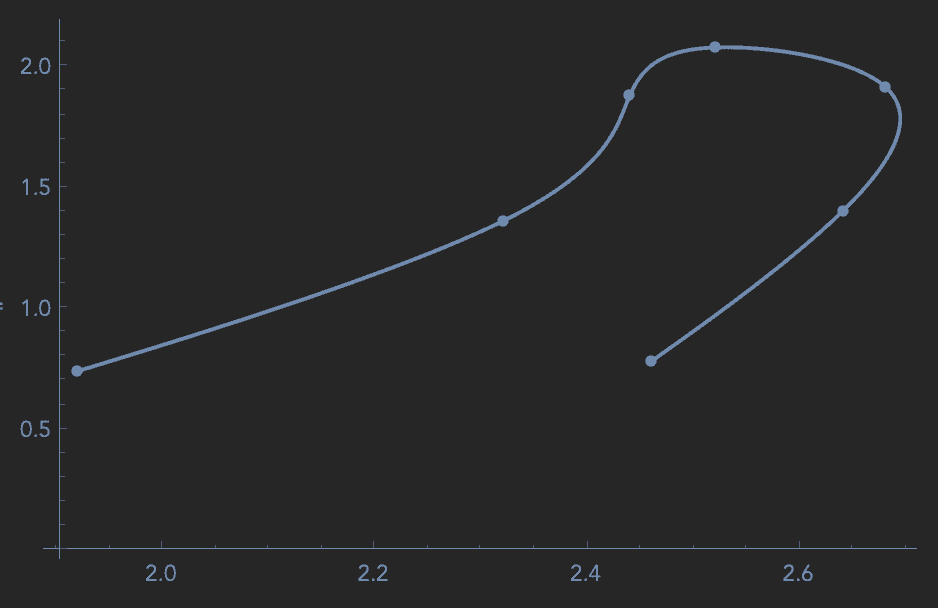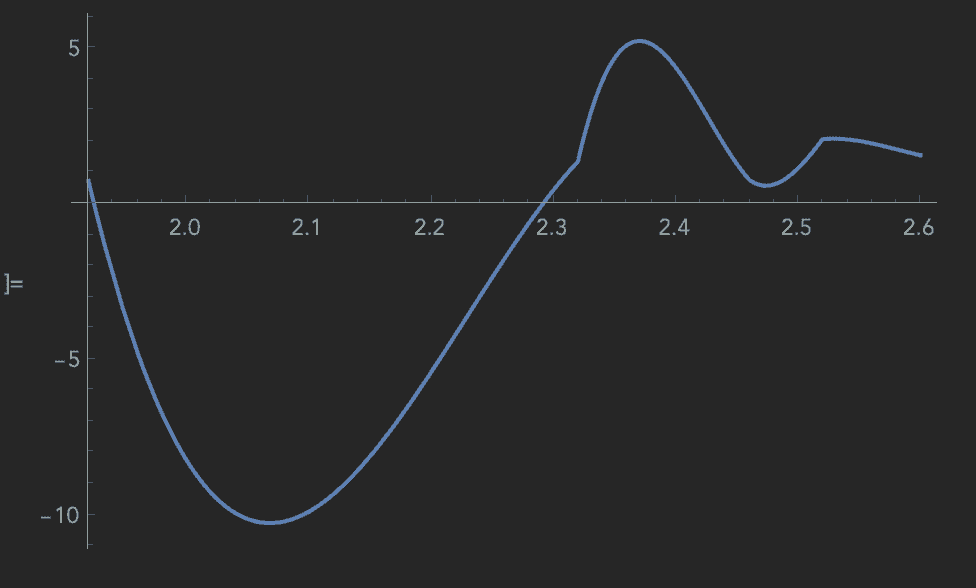# Extract points from an interpolated curve (not a function)

• Mathematica
Gold Member
Hi PF!

I have the given data points here
Code:
data =
{{1.92, 0.74}, {2.32, 1.36}, {2.44, 1.88}, {2.52, 2.08}, {2.68,
1.92}, {2.64, 1.4}, {2.46, 0.78}};

and the following plots the correct interpolation

Code:
Show[{ListLinePlot[{data}, InterpolationOrder -> 3],
ListPlot[\[Lambda]cplx1]}]but I don't know how to extract points along this curve. If I try this

Code:
f = Interpolation[data];
Plot[f[x], {x, 1.92, 2.6}]
the plot is terrible:Any ideas how to extract several points from the correct function?

Mentor
It looks like the data points represent a curve in a 2D space parameterized by a single unspecified parameter. So the points are {x[t],y[t]} with t unspecified.

•joshmccraney
Gold Member
Just thought this exact point! Got it working now, thanks Dale!

•Dale
Mentor
Just thought this exact point! Got it working now, thanks Dale!